# Magnetic Flux

•• Units of magnetic flux are Weber (Wb)

•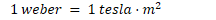••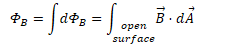# Magnetic Flux Through Closed Surfaces

• Normal to closed surfaces point from the inside to the outside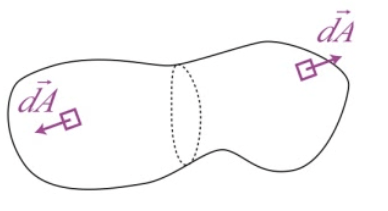# Gauss's Law for Magnetism

• The total magnetic flux through any closed surface is zero

• This would not be true if magnetic monopoles were found to exist

•# Example 1: Flux through a Circular Loop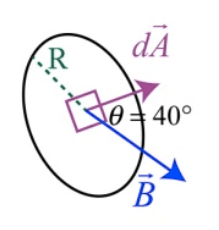• Calculate the flux of 3-Tesla uniform magnetic field through the circular loop of radius 0.2 meters with three turns of wire

•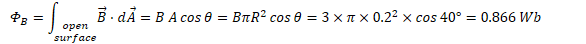# Example 2: Flux Due to a Wire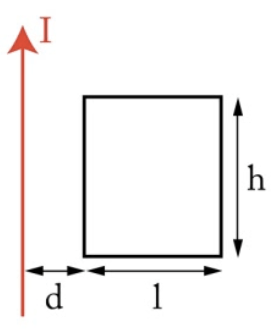• A long straight wire carries a current I as shown.

• Calculate the magnetic flux through the loop

•